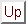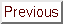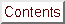Next: A small reminder about Up: Multivariate Lagrange Interpolation Previous: Multivariate Lagrange Interpolation   Contents

# Introduction

One way to generate the local approximation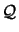of the objective function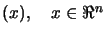around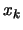is to make Multivariate Lagrange Interpolation.
We will sampleat different points and construct a quadratic polynomial which interpolates these samples.
The position and the numberof the points are not random. For example, if we try to construct a polynomialof degree 1 (a plane), which interpolates locally a function F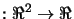, we need exactly 3 pointsand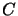. Why do we need exactly 3 points (apart from the fact that 3 points in 3D determines a plane)? Because we need to solve forthe following linear system: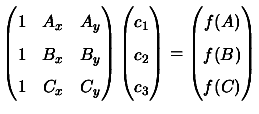(3.1)

The matrix above is called the Vandermonde Matrix''.
We can say even more: What happens if these three points are on the same line? There is a simple infinity of planes which passes through three aligned points. The determinant of the Vandermonde Matrix (called here after the Vandermonde determinant'') will be null. The interpolation problem is not solvable. We will say that ''the problem is NOT poised''.
In opposition to the univariate polynomial interpolation (where we can take a random number of point, at random different places), the multivariate polynomial interpolation imposes a precise number of interpolation points at precise places.
In fact, if we want to interpolate by a polynomial of degree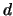a function F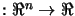, we will need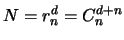points (with). If the Vandermonde determinant is not null for this set of points, the problem is well poised''.
Example: If we want to construct a polynomial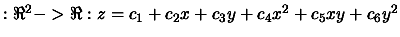of degree 2, which interpolates locally a function F, at points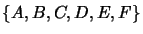we will have the following Vandermonde system: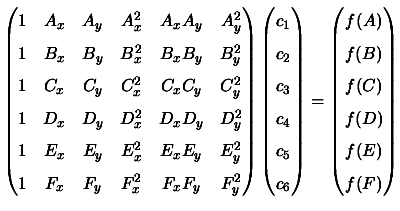Beware! Never try to resolve directly Vandermonde systems. These kind of systems are very often badly conditioned (determinant near zero) and can't be resolved directly.
If we already have a polynomial of degreeand want to use information contained in new points, we will need a block of exactly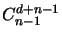new points. The new interpolating polynomial will have a degree of. This is called ''interpolation in block''.Next: A small reminder about Up: Multivariate Lagrange Interpolation Previous: Multivariate Lagrange Interpolation   Contents
Frank Vanden Berghen 2004-04-19